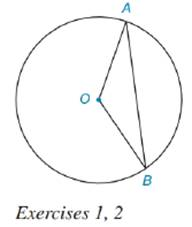Chapter 8.5, Problem 1EElementary Geometry For College St...

7th Edition
Alexander + 2 others
ISBN: 9781337614085

Solutions

Chapter
SectionElementary Geometry For College St...

7th Edition
Alexander + 2 others
ISBN: 9781337614085
Textbook Problem

Given ⊙ O with radii O A - and O B - and chord A B - .a) What type of figure (sector or segment) is bounded by O A - , O B - and A B ^ ?b) If O A = 7 cm and l A B ^ = 11 cm, find the perimeter of the figure in (a).To determine

a. To find:

The type of figure bounded by OA-, OB- and AB^

Explanation

Sector is a pie shaped part of the circle enclosed by two radii and their intercepted arc.

In the given diagram, OA- and OB- are the radii of circle with centre O. AB^ is the arc intercepted by the radii. Thus, the figure bounded by radii OA-, <

To determine

b. To find:

The perimeter of figure in (a).

Still sussing out bartleby?

Check out a sample textbook solution.

See a sample solution

The Solution to Your Study Problems

Bartleby provides explanations to thousands of textbook problems written by our experts, many with advanced degrees!

Get Started

Fill in each blank: 4qt1pt=pt

Elementary Technical Mathematics

Sketch the graph of the function. 48. h(t) = |t| + |t + 1|

Single Variable Calculus: Early Transcendentals

For a given M 0, the corresponding in the definition of limx012x4= is: a) 2M4 b) 2M4 c) 12M4 d) 12M4

Study Guide for Stewart's Single Variable Calculus: Early Transcendentals, 8th

Find the effective rate corresponding to 1058%/year compounded monthly.

Finite Mathematics for the Managerial, Life, and Social Sciences